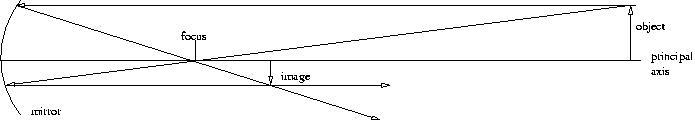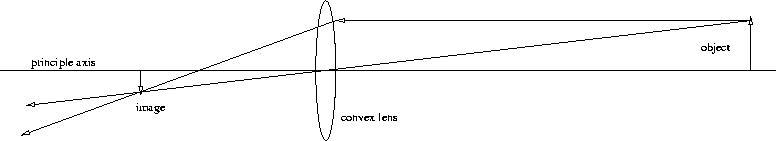# Constructing Optics Diagrams

#### by Robin Riordan

I have noticed that one of the most common questions students have is, “Why does everything look upside down?” So, I thought a little optics review might be in order so that we can all give the questioner a simple graphical answer. .

Drawing a Reflecting Diagram

Let’s start with a concave reflecting surface. There are only two lines you need to draw to locate the image formed by a mirror.

1. Draw a line from the object through the focus and bounce it off the mirror parallel to the principal axis.
2. Draw a line parallel to the principal axis to the mirror and bounce it off through the focusThe point at which the two reflected lines meet is where the image appears. In the diagram above I have drawn the lines just for the top (arrow) of the object. You can construct the rest of the image by picking more points on the object.

The image formed is called a real image. This simply means that the image is formed by lines that are converging to a point.

Drawing a Refracting Diagram

Now, let’s take a look at a refracting telescope. Like a mirror, the lens system has only two line drawing rules.

1. Draw a line from the object parallel to the principal to the lens then refract the line to cross the principal axis.
2. Draw a line straight through the centre of the lens to the refracted line. The image is formed where the two lines meet.The diagrams begin to look complicated when the object is located inside the focus or when you are dealing with a convex mirror or a concave lens because virtual images are formed, but the same construction rules apply.